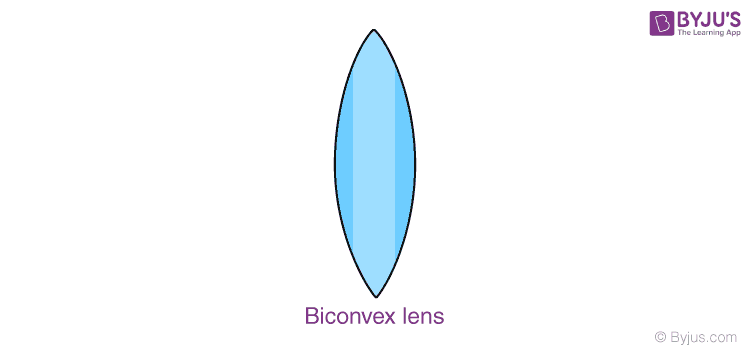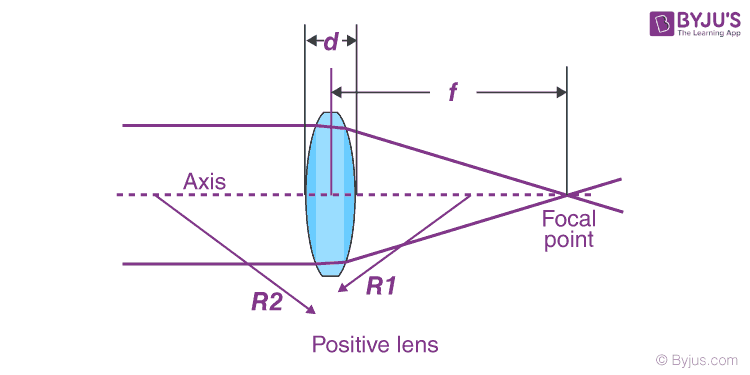# Biconvex Lens

## Biconvex Lenses- Definition and Meaning

Biconvex lens is a simple lens which comprises two convex surfaces in spherical form, generally having the same kind of radius of curvature. These are also called convex-convex lenses.

A Biconvex lens is as shown in the figure below-Being a kind of simple lens, the biconvex lens has a wide range of applications, but it is not limited to control and focusing of laser beams, quality imaging and also the other kinds of optical instruments.

In a biconvex lens or a Plano-convex lens, a collimated or accurately parallel beam of light passes through the lens and converges at a spot or focus coming behind the lens. In such a case, this lens is known as a converging or positive lens. The distance coming from the lens up to the spot is called the focal length of the lens. Since there is a curvature on both sides of the lens, there will be around two focal points and also two centres. The line that is drawn through the middle of the biconvex lens is known as the principal axis. This is as shown in the figure below-A human eye lens is the best example of a Biconvex lens. For an eye, in order to see the objects at hand, the light rays coming from the objects have to bend more sharply in order to bring them on to the focus of the retina; the light rays coming from a distant object require less refraction. To acquire this, it has to be of a highly elastic form so that the shape can be altered and thus can be made less or more convex. The higher the convex lens, the greater the refraction.

### Biconvex Lenses- Properties

Some characteristic properties of a biconvex lens are given below-

• Biconvex lenses are said to possess positive focal length
• They are said to have shorter focal lengths
• They have the ability to converge the incident light
• They have the capability of forming both the real and the virtual images
• They minimize the spherical aberration and can also cancel the coma and the distortion with a conjugate ratio corresponding to unity.

The Biconvex lenses are known to be symmetrical in form, having equal radii on both sides. They are usually recommended for the purpose of virtual imaging in the case of real objects and also for a positive conjugate ratio ranging between 0.2 – 5.

### Biconvex Lenses- Uses & Applications

These lenses have around a number of uses, of which of them are listed below-

1. Biconvex lenses are used as magnifying or condensing lenses.
2. They are also used as objectives or magnifiers
3. These are utilized in many imaging systems such as telescopes, monoculars, microscopes, binoculars, cameras, projectors, etc.
4. These are used in producing the virtual image in case of a human eye and a real image in case of photographic films or an optical sensor
5. These lenses are also used as a burning glass.
6. These are used in the applications of image relays and also in various kinds of industries

Hence, a biconvex lens is one of the best lenses that can be used in a condition where a beam of light incident on a lens is diverging in nature and also the beam that leaves the lenses converges symmetrically.

## Frequently Asked Questions – FAQs

Q1

### What is biconvex lens?

The biconvex lens is a simple lens which consists of two convex surfaces in spherical form, mostly having the same kind of radius of curvature.

Q2

### What is biconvex lens also known as?

The biconvex lens is also known as the convex-convex lens.

Q3

### List three applications of the biconvex lens.

Three applications of the biconvex lens are:
• Biconvex lenses are used as a burning glass.
• They are also used as condensing or magnifying lenses.
• Biconvex lens finds its application in microscopes and binoculars.
• Q4

True.
Q5

### Which is an ideal example of a biconvex lens?

The human eye is an ideal example of a biconvex lens.

## Watch the video and revise all the important concepts in the chapter Light Reflection and Refraction Class 10Stay tuned to BYJU’S and Fall in Love with Learning!

Test your knowledge on Biconvex lens